如果要问在当下的中国，普及速度最快的体育项目是什么，那马拉松一定能排进前三位。

目前在中国田径协会登记在册的各类马拉松赛事已经超过了1000场，这个数字在2010年前后还只有两位数。除了因为高原不适合开展马拉松比赛的西藏，几乎全国所有省区市都有自己的马拉松比赛。

跑步，这个最简单、最容易开展的运动项目，在中国成为了一种覆盖全民的潮流。甚至走在街头，那些体育运动品牌的广告牌上，已经看不到以往那些三大球明星，而只是一位普通的跑者。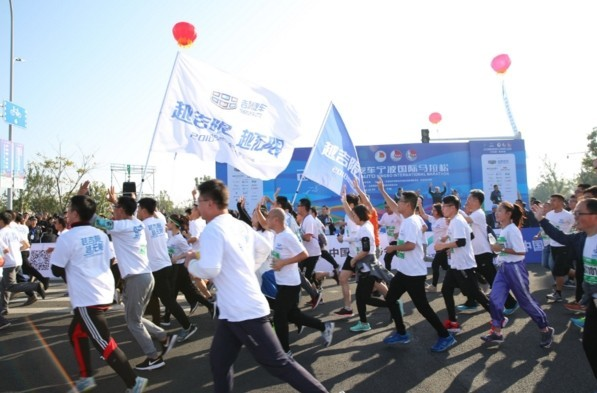马拉松赛事参与人数多，赛事距离长的特点，令其所到之处，都会成为一座城市的全民嘉年华。马拉松赛事的蓬勃兴起，以及人们高涨的参与热情，让这项运动进入了一种良性发展状态。

在刚刚过去的10月28日，2018宁波国际马拉松在宁波杭州湾新区开跑。在这场比赛中，吉利汽车作为冠名赞助商，扮演者特殊的角色。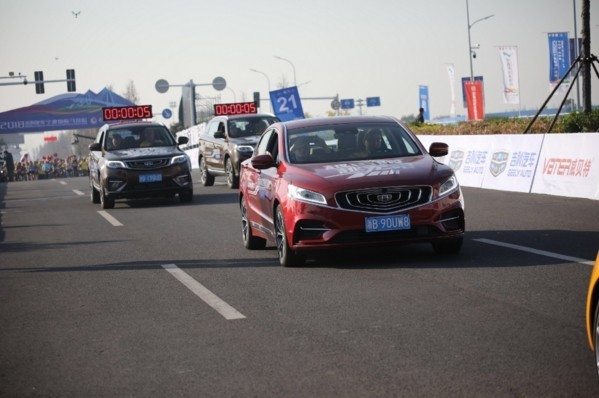与企业通常的赞助方式所不同的是，吉利在甬马的角色不仅仅是赞助商，更是参与者。身为赞助商，赛事期间的保障车辆自然都是吉利出品，包括作为保障车辆的博瑞GE、博越，以及承担领跑车任务的路特斯跑车。

但全场最吸引人的，除了吉利旗下的车型产品，还有一支千人规模的吉利跑团，在这支由吉利员工、经销商、车主组成的队伍中，还出现了李书福、安聪慧等吉利核心管理层的身影。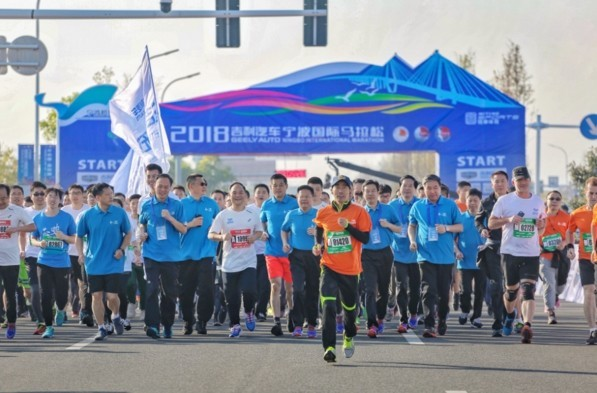熟悉吉利的人都知道，吉利可能是目前国内，在企业内部开展马拉松运动最广泛的企业之一。吉利不仅赞助了宁波国际马拉松、台州国际马拉松等许多马拉松赛事，还主办了吉利帝豪向上马拉松公开赛，在全国各地工作的吉利员工都会就近参与到这些赛事当中。

吉利的这种“马拉松文化”，不止局限于跑步本身，更贯穿于吉利的造车事业中。在李书福、安聪慧等高层领导的讲话中，常常将吉利所从事的造车事业，同马拉松联系起来。跑马拉松，于吉利人而言，不仅是一场锻炼身体愉悦身心的体育运动，更是从每一个向前奔跑的脚印中，汲取着在造车道路上不断向前的精神动力。

李书福董事长曾经这样阐述马拉松运动与造车事业之间的关系：“从事汽车制造业就是一场没有尽头的马拉松，不但需要具备远距离、长时间战斗的体能和技术，更应该拥有马拉松顽强拼搏、永不止步的精神。”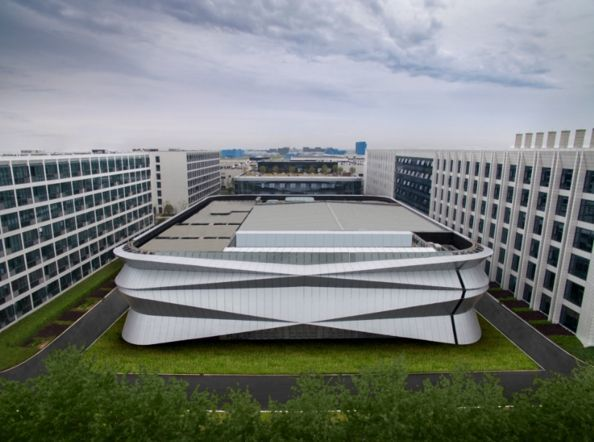今年的甬马比赛路线设在宁波杭州湾新区，途径吉利（杭州湾）研发中心。这里是吉利新技术和新产品的摇篮。在研发中心一期投入运营后，这里还将建设投资额达到70亿元的二期和三期工程，世界领先的安全碰撞试验室、环境风洞实验室和动力新能源实验中心都将在杭州湾落成。

吉利跑团的成员中，有很多来自研发中心的员工，也有不少同在宁波的杭州湾公司、北仑基地、春晓基地的员工。而甬马的参赛选手在比赛过程中，可以近距离直观感受吉利的“智造”实力。这也是吉利23年来在汽车工业领域不断探索不断奋进的缩影。

吉利造车，始于1997年。彼时国内的汽车行业规模远不能和今天相比，汽车在普通民众眼里还属于奢侈品。吉利却以民营企业的身份，率先大胆尝试，喊出“造老百姓买得起的车”。李书福董事长那句“如果失败，请给我一次失败的机会。”在今天看来，可谓饱含着破釜沉舟的决心。

从“老百姓买得起的车”，到“老百姓买得起的好车”，再到“每个人的精品车”。吉利从品牌1.0时代到品牌3.0时代，其间的过程并非一帆风顺。这其中，经历了国内合资车企集中生长的年代，也遭遇过吉利收购沃尔沃初期的外界质疑，更曾有过产品型谱调整的阵痛，但是就像一场马拉松一样，谁能率先冲过42.195公里之外的终点，并不取决于出发时的速度亦或某1公里的冲刺。

马拉松，需要的是坚持，造车亦然。

如果说1997年第一台吉利汽车下线，靠的是破釜沉舟，那么多年间从小到大，由弱变强的过程，就绝不是靠一腔热情所能够达成的。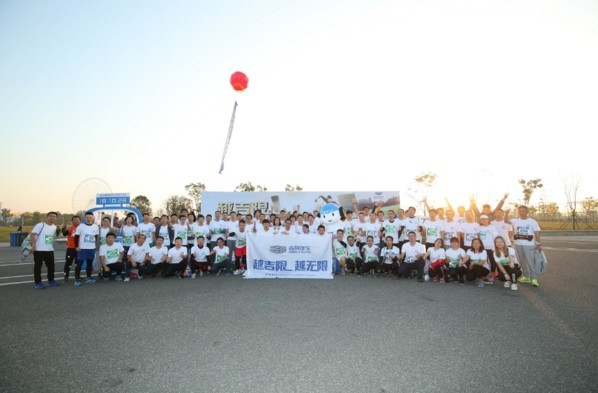人们往往会看到吉利在收购沃尔沃、入股宝腾过程中的果决，却很容易忽略每一次跨出战略性脚步的背后都是长达数年的努力，期间政经大环境、行业竞争格局、企业人事更迭等等这些因素，无时无刻都有可能对最终的结果产生关键影响。就好比在一场马拉松赛跑中，你不知道恶劣的天气、呼吸的极限、肌肉的疲劳会在什么时候突然向你袭来。

应对变化的底气，同样来源于坚持。

近年来随着产品迈入3.0时代，吉利一方面在销量方面取得快速增长，新推出的车型几乎款款热卖，单个车型月销过万几乎成了“起步价”。另一方面，技术研发方面的成果同样层出不穷，开放融合的GKUI吉客智能车联系统、全面发力新能源技术的“智擎”新能源动力系统，越来越多的新技术新科技，正让吉利扮演着中国车企领军者的角色。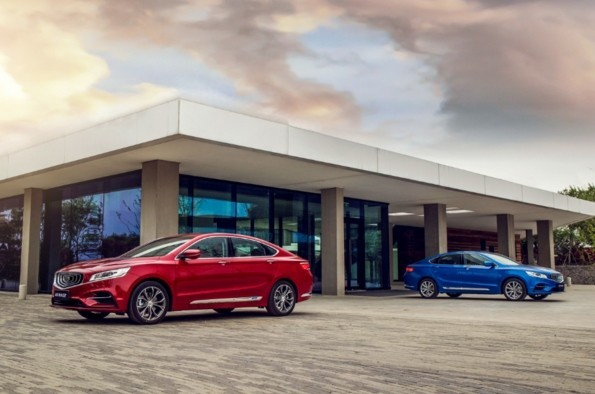但是就像李书福说的那样，造车这场马拉松是没有终点的。坚持，可以让你成为跑道上的强者；坚持，同样是在造车这场马拉松上一直奔跑下去的动力之源。奔跑不息，是吉利的底色。

##### 相关资讯

|分享

•已阅！握爪
•我手滑为你点赞
•128个赞！
•不明觉厉
•阅后既醉
•有钱！任性
•照片太美，我不敢看
•干货！杠杠的
•高大上
•膜拜中！
•请接受我的膝盖
•猫哥/猫妹么么哒
•神吐槽
•我只笑笑不说话
•我想静静
•窒息
##### 资讯相关车系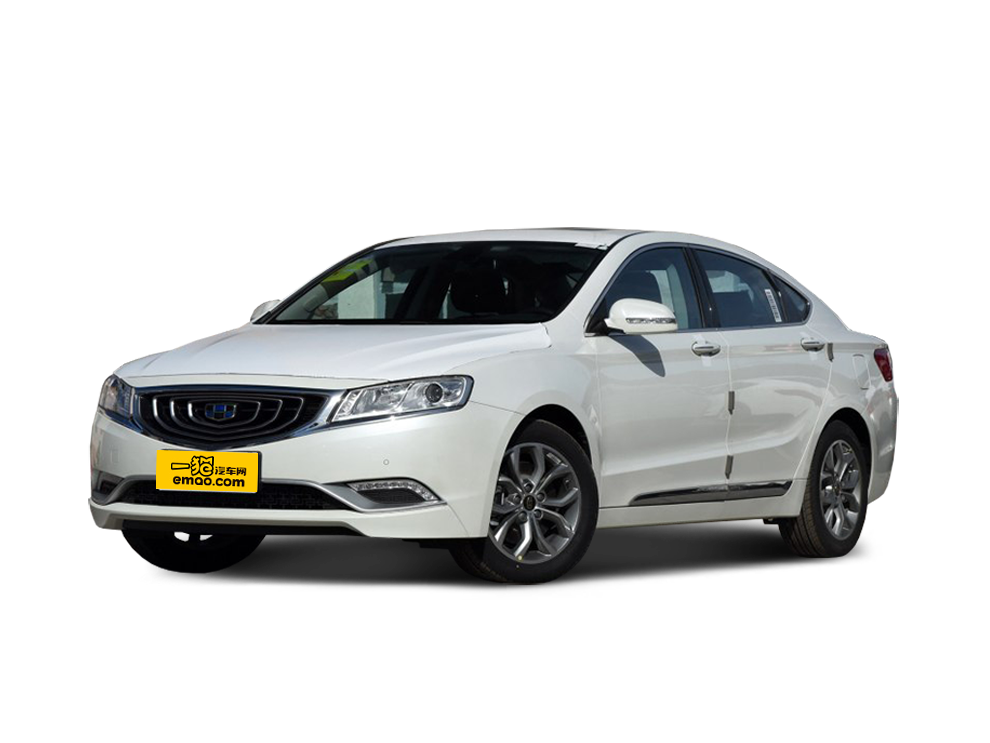##### 热度排行• 阿斯顿·马丁
• 奥迪
• 阿尔法罗密欧
• ALPINA

• 巴博斯
• 宝骏
• 宝马
• 保时捷
• 北汽制造
• 奔驰
• 奔腾
• 本田
• 比亚迪
• 标致
• 别克
• 宾利
• 布加迪
• 北汽威旺
• 北京
• 北汽绅宝
• 北汽幻速
• 北汽新能源
• 宝沃
• 比速汽车
• 北汽道达

• 昌河
• 长安
• 长城
• 长安商用
• 成功汽车
• 长江EV
• 长安轻车型

• 大众
• 道奇
• 东风
• 东风风神
• 东风小康
• 东南
• DS
• 东风风行
• 东风风度
• 东风风光

• 法拉利
• 菲亚特
• 丰田
• 福迪
• 福特
• 福田汽车
• 福汽启腾
• 风诺

• GMC
• 广汽传祺
• 广汽吉奥
• 观致

• 哈飞
• 海格
• 海马
• 华泰
• 黄海
• 恒天
• 红旗
• 哈弗
• 华颂
• 华凯
• 华泰新能源
• 汉腾汽车

• Jeep
• 江淮
• 江铃
• 捷豹
• 金杯
• 九龙
• 吉利汽车
• 金旅
• 金龙
• 江铃集团轻汽
• 江铃集团新能源
• 君马汽车
• 捷途

• 开瑞
• 凯迪拉克
• 科尼赛克
• 克莱斯勒
• KTM
• 卡威
• 凯翼
• 康迪
• 康迪电动汽车集团

• 猎豹汽车
• 兰博基尼
• 劳斯莱斯
• 雷克萨斯
• 雷诺
• 理念
• 力帆
• 莲花汽车
• 林肯
• 铃木
• 陆风
• 路虎
• 路特斯
• 领志
• 领克
• 零跑汽车

• MG
• MINI
• 马自达
• 玛莎拉蒂
• 迈凯伦
• 摩根

• 纳智捷

• 讴歌
• 欧宝
• 欧朗
• 欧拉
• 欧尚汽车

• 帕加尼

• 奇瑞
• 启辰
• 起亚
• 前途
• 庆铃汽车

• 日产
• 荣威
• 瑞麒汽车
• 如虎
• 瑞驰

• 上汽大通
• smart
• 三菱
• 双环
• 双龙
• 斯巴鲁
• 斯柯达
• 萨博
• 思铭
• 赛麟
• SWM斯威汽车

• TESLA
• 泰卡特
• 腾势

• 威麟
• 威兹曼
• 沃尔沃
• 五菱汽车
• 五十铃
• 潍柴英致
• WEY
• 蔚来
• 威马汽车

• 现代
• 雪佛兰
• 雪铁龙
• 西雅特
• 新特汽车
• 小鹏汽车

• 野马汽车
• 一汽
• 依维柯
• 英菲尼迪
• 永源
• 驭胜

• 中华
• 中兴
• 众泰
• 知豆
• 之诺
• 正道汽车
• A
• B
• C
• D
• E
• F
• G
• H
• I
• J
• K
• L
• M
• N
• O
• P
• Q
• R
• S
• T
• U
• V
• W
• X
• Y
• Z

• 阿斯顿·马丁
• 奥迪
• 阿尔法罗密欧
• ALPINA

• 巴博斯
• 宝骏
• 宝马
• 保时捷
• 北汽制造
• 奔驰
• 奔腾
• 本田
• 比亚迪
• 标致
• 别克
• 宾利
• 布加迪
• 北汽威旺
• 北京
• 北汽绅宝
• 北汽幻速
• 北汽新能源
• 宝沃
• 比速汽车
• 北汽道达

• 昌河
• 长安
• 长城
• 长安商用
• 成功汽车
• 长江EV
• 长安轻车型

• 大众
• 道奇
• 东风
• 东风风神
• 东风小康
• 东南
• DS
• 东风风行
• 东风风度
• 东风风光

• 法拉利
• 菲亚特
• 丰田
• 福迪
• 福特
• 福田汽车
• 福汽启腾
• 风诺

• GMC
• 广汽传祺
• 广汽吉奥
• 观致

• 哈飞
• 海格
• 海马
• 华泰
• 黄海
• 恒天
• 红旗
• 哈弗
• 华颂
• 华凯
• 华泰新能源
• 汉腾汽车

• Jeep
• 江淮
• 江铃
• 捷豹
• 金杯
• 九龙
• 吉利汽车
• 金旅
• 金龙
• 江铃集团轻汽
• 江铃集团新能源
• 君马汽车
• 捷途

• 开瑞
• 凯迪拉克
• 科尼赛克
• 克莱斯勒
• KTM
• 卡威
• 凯翼
• 康迪
• 康迪电动汽车集团

• 猎豹汽车
• 兰博基尼
• 劳斯莱斯
• 雷克萨斯
• 雷诺
• 理念
• 力帆
• 莲花汽车
• 林肯
• 铃木
• 陆风
• 路虎
• 路特斯
• 领志
• 领克
• 零跑汽车

• MG
• MINI
• 马自达
• 玛莎拉蒂
• 迈凯伦
• 摩根

• 纳智捷

• 讴歌
• 欧宝
• 欧朗
• 欧拉
• 欧尚汽车

• 帕加尼

• 奇瑞
• 启辰
• 起亚
• 前途
• 庆铃汽车

• 日产
• 荣威
• 瑞麒汽车
• 如虎
• 瑞驰

• 上汽大通
• smart
• 三菱
• 双环
• 双龙
• 斯巴鲁
• 斯柯达
• 萨博
• 思铭
• 赛麟
• SWM斯威汽车

• TESLA
• 泰卡特
• 腾势

• 威麟
• 威兹曼
• 沃尔沃
• 五菱汽车
• 五十铃
• 潍柴英致
• WEY
• 蔚来
• 威马汽车

• 现代
• 雪佛兰
• 雪铁龙
• 西雅特
• 新特汽车
• 小鹏汽车

• 野马汽车
• 一汽
• 依维柯
• 英菲尼迪
• 永源
• 驭胜

• 中华
• 中兴
• 众泰
• 知豆
• 之诺
• 正道汽车
• A
• B
• C
• D
• E
• F
• G
• H
• I
• J
• K
• L
• M
• N
• O
• P
• Q
• R
• S
• T
• U
• V
• W
• X
• Y
• Z

• 阿斯顿·马丁
• 奥迪
• 阿尔法罗密欧
• ALPINA

• 巴博斯
• 宝骏
• 宝马
• 保时捷
• 北汽制造
• 奔驰
• 奔腾
• 本田
• 比亚迪
• 标致
• 别克
• 宾利
• 布加迪
• 北汽威旺
• 北京
• 北汽绅宝
• 北汽幻速
• 北汽新能源
• 宝沃
• 比速汽车
• 北汽道达

• 昌河
• 长安
• 长城
• 长安商用
• 成功汽车
• 长江EV
• 长安轻车型

• 大众
• 道奇
• 东风
• 东风风神
• 东风小康
• 东南
• DS
• 东风风行
• 东风风度
• 东风风光

• 法拉利
• 菲亚特
• 丰田
• 福迪
• 福特
• 福田汽车
• 福汽启腾
• 风诺

• GMC
• 广汽传祺
• 广汽吉奥
• 观致

• 哈飞
• 海格
• 海马
• 华泰
• 黄海
• 恒天
• 红旗
• 哈弗
• 华颂
• 华凯
• 华泰新能源
• 汉腾汽车

• Jeep
• 江淮
• 江铃
• 捷豹
• 金杯
• 九龙
• 吉利汽车
• 金旅
• 金龙
• 江铃集团轻汽
• 江铃集团新能源
• 君马汽车
• 捷途

• 开瑞
• 凯迪拉克
• 科尼赛克
• 克莱斯勒
• KTM
• 卡威
• 凯翼
• 康迪
• 康迪电动汽车集团

• 猎豹汽车
• 兰博基尼
• 劳斯莱斯
• 雷克萨斯
• 雷诺
• 理念
• 力帆
• 莲花汽车
• 林肯
• 铃木
• 陆风
• 路虎
• 路特斯
• 领志
• 领克
• 零跑汽车

• MG
• MINI
• 马自达
• 玛莎拉蒂
• 迈凯伦
• 摩根

• 纳智捷

• 讴歌
• 欧宝
• 欧朗
• 欧拉
• 欧尚汽车

• 帕加尼

• 奇瑞
• 启辰
• 起亚
• 前途
• 庆铃汽车

• 日产
• 荣威
• 瑞麒汽车
• 如虎
• 瑞驰

• 上汽大通
• smart
• 三菱
• 双环
• 双龙
• 斯巴鲁
• 斯柯达
• 萨博
• 思铭
• 赛麟
• SWM斯威汽车

• TESLA
• 泰卡特
• 腾势

• 威麟
• 威兹曼
• 沃尔沃
• 五菱汽车
• 五十铃
• 潍柴英致
• WEY
• 蔚来
• 威马汽车

• 现代
• 雪佛兰
• 雪铁龙
• 西雅特
• 新特汽车
• 小鹏汽车

• 野马汽车
• 一汽
• 依维柯
• 英菲尼迪
• 永源
• 驭胜

• 中华
• 中兴
• 众泰
• 知豆
• 之诺
• 正道汽车
• A
• B
• C
• D
• E
• F
• G
• H
• I
• J
• K
• L
• M
• N
• O
• P
• Q
• R
• S
• T
• U
• V
• W
• X
• Y
• Z